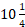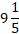• Save

# Time and Work Short Tricks Solved Problems for Competitive Exams

Last month 12.1K ViewsTime and Work is an important topic in the Quantitative Aptitude section for Bank and SSC Exam. In this topic, 2 to 3 questions are asked every year in competition exam. You should learn the shortcut tricks for saving time in the competitive exams.

Here is a detailed introduction of Time and Work along with short tricks which will help the candidates to prepare the topic well. For more practice, you can visit on Time and work problems with solutions.

Here you can get all the topics of Time and Work Aptitude by click here.

## What is Time and work?

Performing or doing any work involves efforts of person over a period of time. Therefore, the number of person (P), the quantity of work (W) and the period of time taken (T) are important variables in problem-related to time and work.

## Time and Work Short Tricks Solved Problems

Example 1: Chandni and Divakar can do a piece of work in 9 days and 12 days, respectively. If they work for a day alternatively, Chandni beginning in how many days, the work will be completed?

(A)(B)(C) 11.11

(D) 10

Solution

. Chandni’s 1 days’s work = 1/9.

Divakar’s 1 day’s work = 1/12.

Chandni and Divakar’s (1+1) = 2.

Day’s work = (1/9)+(1/12) = 7/36

So, in 10 days they do = (7×5)/36 = 35/36.

So, the remaining 1/36 {= 1-35/36}

Work will be done by Chandni

= (1/36)/(1/9) = 1/4.

Thus, total number of required days

= 10+(1/4) =days

Short Tricks

Efficiency of Chandni = 11.11%

Efficiency of Divakar = 8.33%

They do 19.44% work in 2 days.

⸫  They need 10 days to do 97.22% work.

Now, the rest work (2.78) was done by Chandni in 2.78/11.11 = 1/4 day

Therefore, total number of days

Required = 10+1/4 =Example 2: Pipe A can fill an empty tank in 30 h while B can fill it in 45 h. Pipe A and B are opened and closed alternatively i.e., first pipe A is opened, then B, again A and then B and so on for 1 h each time without any time lapse. In how many hours the tank will be filled when it was empty, initially?

(A) 36

(B) 54

(C) 48

(D) 60

Solution

In 1 h pipe A can fill = 1/30 part of the tank

In 1 h pipe B can fill = 1/45 part of the tank

In 2 h pipes A and B can fill = 1/18 part of the tank

Therefore, in 36 h the tank will be completely filled.

Short Tricks

Efficiency of pipe A = 3.33%

Efficiency of pipe B = 2.22%

And combined efficiency = 5.55%

Therefore, in 2 h pipes A and B fill 5.55%. Thus, to fill 100% tank, these pipes will take 36 h.

Example 3: If 20 persons can do a piece of work in 7 days, then calculate the number of persons required to complete the work in 28 days.

(A) 5

(B) 10

(C) 15

(D) 25

Solution

Number of persons × days = work.

20 × 7 = 140 man-days.

Now, X × 28 = 140 man-days.

→ X = 5

Therefore, in second case the required number of person is 5.

Short Tricks

Since, work in constant, therefore

M1×D1 = M2×D2 = Work done

20 × 7 = M2 × 28 → M2 = 5.

Example 4: A can do a piece of work in 14 days, while B can do it in 21 days. In how many days, working together they will complete the whole work?

(A) 10.5

(B) 8

(C) 8.4

(D) 9

Solution

Efficiency of A = 7.14%

Efficiency of B = 4.76%

Efficiency of (A+B) = 11.9%

⸫ Number of days required by A and B, working together = 100/11.9 = 8.4 days

Hint you can see, that there is only one option between 8 and 9, which is 8.4.

For 8 days denominator should be 12.5 as for 9 days denominator should be almost 11.

Short Trick

1 days work of A and B = 1/14 + 1/21 = 5/42

⸫ Required number of days = 42/5 = 8.4 days.

Example 5: 16 men finished one-third work in 6 days. The number of additional men are required to complete the job in next 6 days.

(A) 10

(B) 8

(C) 16

(D) 32

Solution

M × D = W,

16 × 6 = 1/3 W

Rest work = 2/3 W

For double work in same time 32 men are required to do the work or 16 more men required to do the work.

Shor trick

2×(16×6) = 6×M → M = 32.

⸫ 16 more men are required.

Example 6: A group of men decided to do a job in 4 days. But since 20 men dropped out every day, the job completed the at the end of the 7th day. How many men were there at the beginning?

(A) 240

(B) 140

(C) 280

(D) 150

Let n be the initial number of men, then n × 4 = n +(n-20)+(n-40)+…….+(n-120)

→ 4n = 7n – 240 → 3n = 420

⸫ n = 140 men.

Short Tricks

Go through option

140 × 4 = ( 140 + 120 +100+….+20)

560 = 560

Example 7: A is twice as good a workman as B and therefore A takes 6 days less than B to finish the work individually. If A and B working together complete the work in 4 days, then how many days are required by B to complete the work alone?

(A) 12

(B) 18

(C) 8

(D) 6

Solution

A      B

Efficiency  →  2 :     1

Days           →  1 :    2

{Days α 1/Efficiency}

Now, let A requires X days, then B requires 2X days

⸫ Difference in number of days

{=(2X – X) = X = 6 → X = 6

⸫ B requires 2X = 2×6 = 12 days.

### Second Method:

If A takes X days, then B takes ( X+6) days.

Now, A’s 1 day’s work = 1/X.

B’s 1 day’s work = 1/(X+6) ⸫ (1/X)/1/(X+6) = 2/1.

(Since, A does twice the work as B does)

→ X = 6

⸫ B takes 2x = 12 days.

### Third Method:

(1/X) + {1/(X+6)} = 1/4.

→ X = 6 and 2X = 12 days (required by B).

Visit on the next page for more practice of these questions. You can ask me anything in the comment section about this topic.